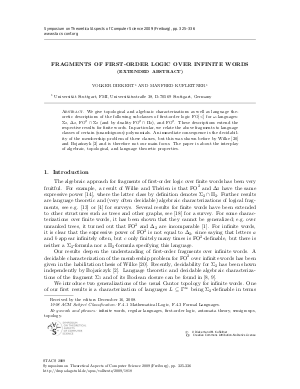Document# Fragments of First-Order Logic over Infinite Words

### Authors Volker Diekert, Manfred Kufleitner## File

LIPIcs.STACS.2009.1818.pdf
• Filesize: 184 kB
• 12 pages

## Cite As

Volker Diekert and Manfred Kufleitner. Fragments of First-Order Logic over Infinite Words. In 26th International Symposium on Theoretical Aspects of Computer Science. Leibniz International Proceedings in Informatics (LIPIcs), Volume 3, pp. 325-336, Schloss Dagstuhl - Leibniz-Zentrum für Informatik (2009)
https://doi.org/10.4230/LIPIcs.STACS.2009.1818

## Abstract

We give topological and algebraic characterizations as well as language theoretic descriptions of the following subclasses of first-order logic $\mathrm{FO}[<]$ for $\omega$-languages: $\Sigma_2$, $\Delta_2$, $\mathrm{FO}^2 \cap \Sigma_2$ (and by duality $\mathrm{FO}^2 \cap \Pi_2$), and $\mathrm{FO}^2$. These descriptions extend the respective results for finite words. In particular, we relate the above fragments to language classes of certain (unambiguous) polynomials. An immediate consequence is the decidability of the membership problem of these classes, but this was shown before by Wilke (1998) and Boja{\'n}czyk (2008) and is therefore not our main focus. The paper is about the interplay of algebraic, topological, and language theoretic properties.
##### Keywords
• Infinite words
• Regular languages
• First-order logic
• Automata theory
• Semigroups
• Topology

## Metrics

• Access Statistics
• Total Accesses (updated on a weekly basis)
0